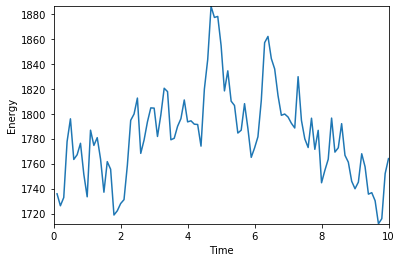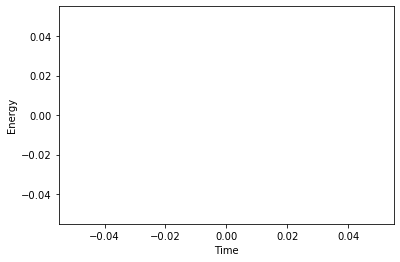# Tutorial 8: Visualization¶

## Introduction¶

When you are running a simulation, it is often useful to see what is going on by visualizing particles in a 3D view or by plotting observables over time. That way, you can easily determine things like whether your choice of parameters has led to a stable simulation or whether your system has equilibrated. You may even be able to do your complete data analysis in real time as the simulation progresses.

Thanks to ESPResSo's Python interface, we can make use of standard libraries like Mayavi or OpenGL (for interactive 3D views) and Matplotlib (for line graphs) for this purpose. We will also use NumPy, which both of these libraries depend on, to store data and perform some basic analysis.

## Simulation¶

First, we need to set up a simulation. We will simulate a simple Lennard-Jones liquid.

In :
from matplotlib import pyplot

In :
import espressomd
import numpy

espressomd.assert_features("LENNARD_JONES")

# system parameters (10000 particles)
box_l = 10.7437
density = 0.7

# interaction parameters (repulsive Lennard-Jones)
lj_eps = 1.0
lj_sig = 1.0
lj_cut = 1.12246
lj_cap = 20

# integration parameters
system = espressomd.System(box_l=[box_l, box_l, box_l])
system.time_step = 0.0001
system.cell_system.skin = 0.4
system.thermostat.set_langevin(kT=1.0, gamma=1.0, seed=42)

# warmup integration (with capped LJ potential)
warm_steps = 100
warm_n_times = 30
# do the warmup until the particles have at least the distance min_dist
min_dist = 0.9

# integration
int_steps = 1000
int_n_times = 100

#############################################################
#  Setup System                                             #
#############################################################

# interaction setup
system.non_bonded_inter[0, 0].lennard_jones.set_params(
epsilon=lj_eps, sigma=lj_sig,
cutoff=lj_cut, shift="auto")
system.force_cap = lj_cap

# particle setup
volume = box_l * box_l * box_l
n_part = int(volume * density)
for i in range(n_part):

act_min_dist = system.analysis.min_dist()

#############################################################
#  Warmup Integration                                       #
#############################################################

# set LJ cap
lj_cap = 20
system.force_cap = lj_cap

# warmup integration loop
i = 0
while (i < warm_n_times and act_min_dist < min_dist):
system.integrator.run(warm_steps)
# warmup criterion
act_min_dist = system.analysis.min_dist()
i += 1
# increase LJ cap
lj_cap = lj_cap + 10
system.force_cap = lj_cap

#############################################################
#      Integration                                          #
#############################################################

# remove force capping
lj_cap = 0
system.force_cap = lj_cap

def main():
for i in range(int_n_times):
print("\rrun %d at time=%.0f " % (i, system.time), end='')
system.integrator.run(int_steps)
print('\rSimulation complete')

main()

Simulation complete


## Live plotting¶

Let's have a look at the total energy of the simulation. We can determine the individual energies in the system using system.analysis.energy(). We will adapt the main() function to store the total energy at each integration run into a NumPy array. We will also create a function to draw a plot after each integration run.

In :
matplotlib_notebook = True  # toggle this off when outside IPython/Jupyter

# setup matplotlib canvas
pyplot.xlabel("Time")
pyplot.ylabel("Energy")
plot, = pyplot.plot(, )
if matplotlib_notebook:
from IPython import display
else:
pyplot.show(block=False)

# setup matplotlib update function
current_time = -1
def update_plot():
i = current_time
if i < 3:
return None
plot.set_xdata(energies[:i + 1, 0])
plot.set_ydata(energies[:i + 1, 1])
pyplot.xlim(0, energies[i, 0])
pyplot.ylim(energies[:i + 1, 1].min(), energies[:i + 1, 1].max())
# refresh matplotlib GUI
if matplotlib_notebook:
display.clear_output(wait=True)
display.display(pyplot.gcf())
else:
pyplot.draw()
pyplot.pause(0.01)

# re-define the main() function
def main():
global current_time
for i in range(int_n_times):
system.integrator.run(int_steps)
energies[i] = (system.time, system.analysis.energy()['total'])
current_time = i
update_plot()
if matplotlib_notebook:
display.clear_output(wait=True)

system.time = 0  # reset system timer
energies = numpy.zeros((int_n_times, 2))
main()

if not matplotlib_notebook:
pyplot.close()## Live visualization and plotting¶

To interact with a live visualization, we need to move the main integration loop into a secondary thread and run the visualizer in the main thread (note that visualization or plotting cannot be run in secondary threads). First, choose a visualizer:

In :
from espressomd import visualization

visualizer = visualization.openGLLive(system)
# alternative: visualization.mayaviLive(system)


Then, re-define the main() function to run the visualizer:

In :
def main():
global current_time
for i in range(int_n_times):
system.integrator.run(int_steps)
energies[i] = (system.time, system.analysis.energy()['total'])
current_time = i
visualizer.update()

system.time = 0  # reset system timer


Next, create a secondary thread for the main() function. However, as we now have multiple threads, and the first thread is already used by the visualizer, we cannot call update_plot() from the main() anymore. The solution is to register the update_plot() function as a callback of the visualizer:

In :
# setup new matplotlib canvas
if matplotlib_notebook:
pyplot.xlabel("Time")
pyplot.ylabel("Energy")
plot, = pyplot.plot(, )

# execute main() in a secondary thread
t.daemon = True
t.start()

# execute the visualizer in the main thread
visualizer.register_callback(update_plot, interval=int_steps // 2)
visualizer.start()

Out:
<MagicMock name='mock.openGLLive().start()' id='140287742905648'>While the simulation is running, you can move and zoom around with your mouse.

Important: closing the visualizer GUI will exit the Python session!

If the trajectory runs too quickly, try decreasing int_steps by a factor 10.

You can try Mayavi by switching the visualizer to visualization.mayaviLive(system). In Mayavi, explore the buttons in the toolbar to see how the graphical representation can be changed.

### Alternative: live visualization without plotting¶

If live plotting is not important, the code in the previous section simplifies to:

from espressomd import visualization
visualizer = visualization.openGLLive(system)

def main():
for i in range(int_n_times):
system.integrator.run(int_steps)
energies[i] = (system.time, system.analysis.energy()['total'])
visualizer.update()

system.time = 0  # reset system timer

# execute main() in a secondary thread
t.daemon = True
t.start()
visualizer.start()


### Alternative: live visualization only¶

If recording the energy as a time series is not important, the code in the previous section simplifies even further:

from espressomd import visualization
visualizer = visualization.openGLLive(system)
visualizer.run()


## Customizing the OpenGL visualizer¶

Visualization of more advanced features of ESPResSo is also possible (e.g. bonds, constraints, lattice-Boltzmann) with the OpenGL visualizer. There are a number of optional keywords that can be used to specify the appearance of the visualization, they are simply stated after system when creating the visualizer instance. See the following examples:

# Enables particle dragging via mouse:
visualizer = visualization.openGLLive(system, drag_enabled=True)

# Use a white background:
visualizer = visualization.openGLLive(system, background_color=[1, 1, 1])

# Use red color for all (uncharged) particles
visualizer = visualization.openGLLive(system, particle_type_colors=[[1, 0, 0]])


The visualizer options are stored in the dict visualizer.specs. The following snippet prints out the current configuration nicely:

for k in sorted(visualizer.specs.keys(), key=lambda s: s.lower()):
print("{:30}  {}".format(k, visualizer.specs[k]))


All keywords are explained in the Online Documentation at http://espressomd.org/html/doc/visualization.html#opengl-visualizer. Specific visualization examples for ESPResSo can be found in the /samples folder. You may need to recompile ESPResSo with the required features used in the examples.